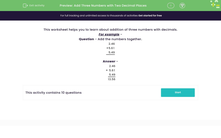# Add Three Numbers with Two Decimal Places

In this worksheet, students add together three numbers, each of which has two decimal places.Key stage:  KS 2

Curriculum topic:   Number: Fractions and Decimals

Curriculum subtopic:   Solve Fraction and Decimal Problems

Difficulty level:#### Worksheet Overview

This worksheet helps you to learn about addition of three numbers with decimals.

For example -

Question - Add the numbers together.

Add the columns, starting from the right-hand column.

Remember:

• Keep the columns lined up correctly
• Carry over when you cross 10
• The decimal place does not move
• Look for bonds to 10 to add first  e.g. 9 + 1 = 10 + 6 = 16
 + 2 . 4 6 5 . 6 1 5 . 4 9